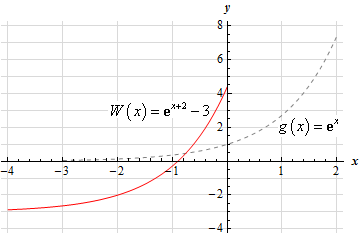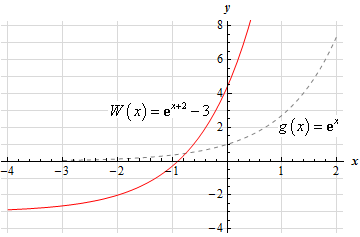Paul's Online Notes
Home / Calculus I / Review / Common Graphs
Show Mobile Notice Show All Notes Hide All Notes
Mobile Notice
You appear to be on a device with a "narrow" screen width (i.e. you are probably on a mobile phone). Due to the nature of the mathematics on this site it is best views in landscape mode. If your device is not in landscape mode many of the equations will run off the side of your device (should be able to scroll to see them) and some of the menu items will be cut off due to the narrow screen width.

### Section 1.10 : Common Graphs

7. Without using a graphing calculator sketch the graph of $$W\left( x \right) = {{\bf{e}}^{x + 2}} - 3$$.

Hint : The Algebraic transformations that we used to help us graph the first few graphs in this section can be used together to shift the graph of a function both up/down and right/left at the same time.
Show Solution

The Algebraic transformations we were using in the first few problems of this section can be combined to shift a graph up/down and right/left at the same time. If we know the graph of $$g\left( x \right)$$ then the graph of $$g\left( {x + c} \right) + k$$ is simply the graph of $$g\left( x \right)$$ shifted right by $$c$$ units if $$c < 0$$ or shifted left by $$c$$ units if $$c > 0$$ and shifted up by $$k$$ units if $$k > 0$$ or shifted down by $$k$$ units if $$k < 0$$.

So, in our case if $$g\left( x \right) = {{\bf{e}}^x}$$ we can see that,

$W\left( x \right) = {{\bf{e}}^{x + 2}} - 3 = g\left( {x + 2} \right) - 3$

and so the graph we’re being asked to sketch is the graph of $$g\left( x \right) = {{\bf{e}}^x}$$shifted left by 2 units and down by 3 units.

Here is the graph of $$W\left( x \right) = {{\bf{e}}^{x + 2}} - 3$$ and note that to help see the transformation we have also sketched in the graph of $$g\left( x \right) = {{\bf{e}}^x}$$.In this case the resulting sketch of $$W\left( x \right)$$ that we get by shifting the graph of $$g\left( x \right)$$ is not really the best, as it pretty much cuts off at $$x = 0$$ so in this case we should probably extend the graph of $$W\left( x \right)$$ a little. Here is a better sketch of the graph.